UPSC  >  Test: Square Root And Cube Root- 2

# Test: Square Root And Cube Root- 2 - UPSC

Test Description

## 10 Questions MCQ Test CSAT Preparation - Test: Square Root And Cube Root- 2

Test: Square Root And Cube Root- 2 for UPSC 2023 is part of CSAT Preparation preparation. The Test: Square Root And Cube Root- 2 questions and answers have been prepared according to the UPSC exam syllabus.The Test: Square Root And Cube Root- 2 MCQs are made for UPSC 2023 Exam. Find important definitions, questions, notes, meanings, examples, exercises, MCQs and online tests for Test: Square Root And Cube Root- 2 below.
Solutions of Test: Square Root And Cube Root- 2 questions in English are available as part of our CSAT Preparation for UPSC & Test: Square Root And Cube Root- 2 solutions in Hindi for CSAT Preparation course. Download more important topics, notes, lectures and mock test series for UPSC Exam by signing up for free. Attempt Test: Square Root And Cube Root- 2 | 10 questions in 20 minutes | Mock test for UPSC preparation | Free important questions MCQ to study CSAT Preparation for UPSC Exam | Download free PDF with solutions
 1 Crore+ students have signed up on EduRev. Have you?
Test: Square Root And Cube Root- 2 - Question 1

### Practice Quiz or MCQ (Multiple Choice Questions) with solutions are available for Practice, which would help you prepare for "Square Root and Cube Root" under Quantitative Aptitude. You can practice these practice quizzes as per your speed and improvise the topic. The same topic is covered under various competitive examinations like - CAT, GMAT, Bank PO, SSC and other competitive examinations.   Q. The cube root of .000216 is:

Detailed Solution for Test: Square Root And Cube Root- 2 - Question 1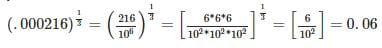Test: Square Root And Cube Root- 2 - Question 2

### What should come in place of both x in the equation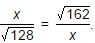Detailed Solution for Test: Square Root And Cube Root- 2 - Question 2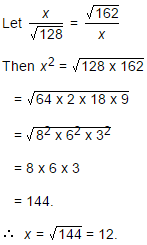Test: Square Root And Cube Root- 2 - Question 3

### The least perfect square, which is divisible by each of 21, 36 and 66 is:

Detailed Solution for Test: Square Root And Cube Root- 2 - Question 3

L.C.M. of 21, 36, 66 = 2772.

Now, 2772 = 2 x 2 x 3 x 3 x 7 x 11

To make it a perfect square, it must be multiplied by 7 x 11.

So, required number = 22 x 32 x 72 x 112 = 213444

Test: Square Root And Cube Root- 2 - Question 4

What is the Square Root of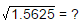Detailed Solution for Test: Square Root And Cube Root- 2 - Question 4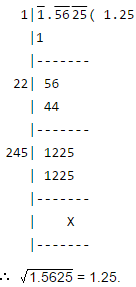Test: Square Root And Cube Root- 2 - Question 5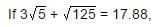then what will be the value of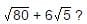Detailed Solution for Test: Square Root And Cube Root- 2 - Question 5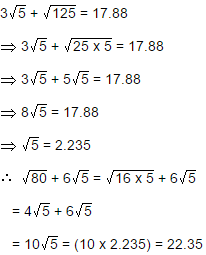Test: Square Root And Cube Root- 2 - Question 6

If a = 0.1039, then the value of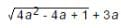is:

Test: Square Root And Cube Root- 2 - Question 7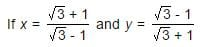then the value of (x2 + y2) is:

Detailed Solution for Test: Square Root And Cube Root- 2 - Question 7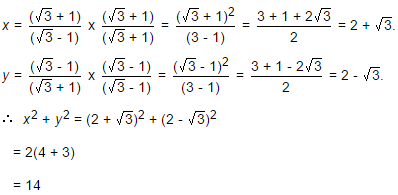Test: Square Root And Cube Root- 2 - Question 8

A group of students decided to collect as many paise from each member of group as is the number of members. If the total collection amounts to Rs. 59.29, the number of the member is the group is:

Detailed Solution for Test: Square Root And Cube Root- 2 - Question 8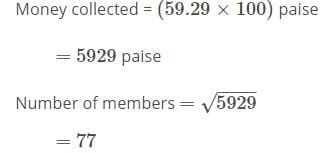Test: Square Root And Cube Root- 2 - Question 9

The square root of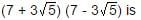Detailed Solution for Test: Square Root And Cube Root- 2 - Question 9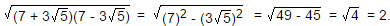Test: Square Root And Cube Root- 2 - Question 10

If √5 = 2.236, then the value of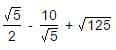is equal to:

Detailed Solution for Test: Square Root And Cube Root- 2 - Question 10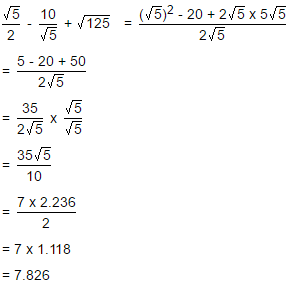## CSAT Preparation

197 videos|151 docs|200 tests
Information about Test: Square Root And Cube Root- 2 Page
In this test you can find the Exam questions for Test: Square Root And Cube Root- 2 solved & explained in the simplest way possible. Besides giving Questions and answers for Test: Square Root And Cube Root- 2, EduRev gives you an ample number of Online tests for practice

## CSAT Preparation

197 videos|151 docs|200 tests

### How to Prepare for UPSC

Read our guide to prepare for UPSC which is created by Toppers & the best Teachers